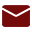## Ü DO (Do)

·         The Do-EndDo loop is used when we have to iterate over a block of statements for specific number (say 10, 20 100) of times.

·         The do loop takes arguments as below:
The Starting Counter, The maximum value and the index value.

·         We can specify any of the above values as variables. The following examples will clarify this loop further.

 Factor 1 Op-code Factor 2 Result Field Resulting Indicators        HI                       LO                      EQ Loop starting index DO Loop ending index Current loop index

Example

```
DCount          S              2P 0 Inz(2)
DValue         S              2P 0
**
C     Count       Do        6             Value
C     Value      Dsply
C                   EndDo
**
C                   Return
```

OUTPUT

DSPLY   2

DSPLY   3

DSPLY   4

DSPLY   5

DSPLY   6

In the above example, the Count is the starting index and 6 is the maximum value of index. This means that the loop will be executed for 6-Count times. Also, if the starting index value (Count) is not specified, the default starting index is taken as 1. The value of the index at any time is stored in the Value. This result field is also optional. If you need this counter then define it otherwise do not.